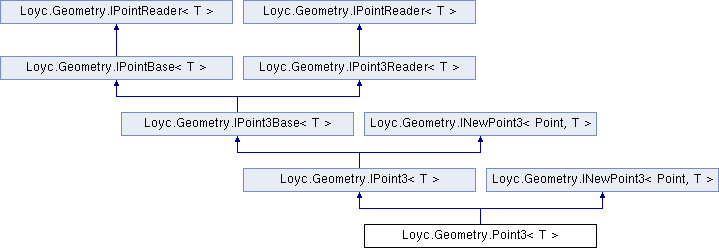Enhanced C# Language of your choice: library documentation

# Documentation moved to ecsharp.net

GitHub doesn't support HTTP redirects, so you'll be redirected in 3 seconds.

Loyc.Geometry.Point3< T > Struct Template Reference

A 3D point (X-Y-Z triplet) structure. More...

Source file:
Inheritance diagram for Loyc.Geometry.Point3< T >:## Remarks

A 3D point (X-Y-Z triplet) structure.

Template Parameters
 T Coordinate type.
PointMath, Vector3{T}
Type Constraints
 T : IConvertible T : IEquatable

_x

_y

_z

## Public static fields

static IMath< T > m = Maths<T>.Math

static readonly Point3< T > Zero = new Point3<T>()

static readonly Point3< T > Inf = new Point3<T>(m.PositiveInfinity, m.PositiveInfinity, m.PositiveInfinity)

## Properties

X` [get, set]`

Y` [get, set]`

Z` [get, set]`Properties inherited from Loyc.Geometry.IPoint3Base< T >
new T Z` [get, set]`
Z coordinate of a point or vector. More...Properties inherited from Loyc.Geometry.IPointBase< T >
new T X` [get, set]`
Horizontal coordinate of a point or vector. More...

new T Y` [get, set]`
Vertical coordinate of a point or vector. More...Properties inherited from Loyc.Geometry.IPointReader< T >
X` [get]`

Y` [get]`Properties inherited from Loyc.Geometry.IPoint3Reader< T >
Z` [get]`

## Public Member Functions

Point3 (T x, T y)

Point3 (T x, T y, T z)

override bool Equals (object other)

override int GetHashCode ()

override string ToString ()

Point3< T > New (T x, T y, T z)

IPoint3< T > INewPoint3
< IPoint3< T >, T >.
New (T x, T y, T z)

bool Equals (Point3< T > other)

LineSegment3< T > To (Point3< T > other)

## Static Public Member Functions

static operator Vector3< T > (Point3< T > p)

static operator Point3< int > (Point3< T > p)

static operator Point3< long > (Point3< T > p)

static operator Point3< float > (Point3< T > p)

static operator Point3< double > (Point3< T > p)

static Point3< T > operator+ (Point3< T > a, Vector3< T > b)

static Point3< T > operator+ (Vector3< T > a, Point3< T > b)

static Point3< T > operator- (Point3< T > a, Vector3< T > b)

static Vector3< T > operator- (Point3< T > a, Point3< T > b)

static Point3< T > operator* (Point3< T > p, T factor)

static Point3< T > operator/ (Point3< T > p, T factor)

static Point3< T > operator<< (Point3< T > p, int amt)

static Point3< T > operator>> (Point3< T > p, int amt)

static bool operator== (Point3< T > a, Point3< T > b)

static bool operator!= (Point3< T > a, Point3< T > b)